Ex 4.6

Chapter 4 Class 12 Determinants (Term 1)
Serial order wise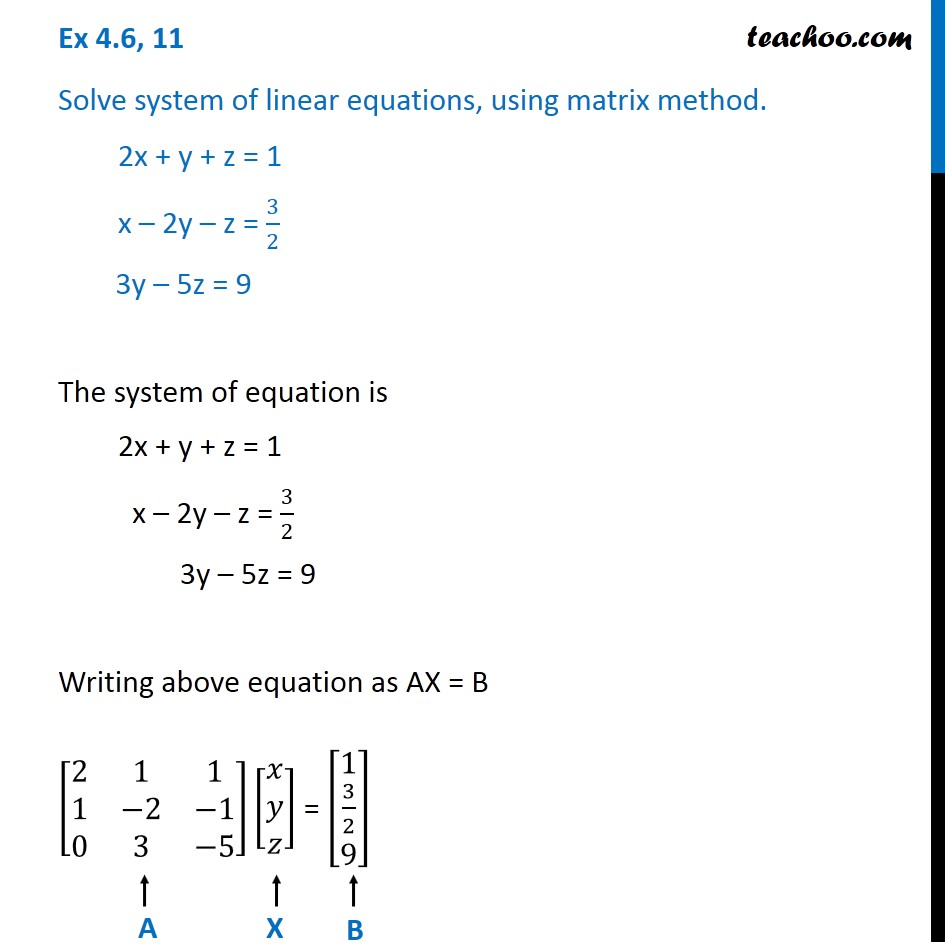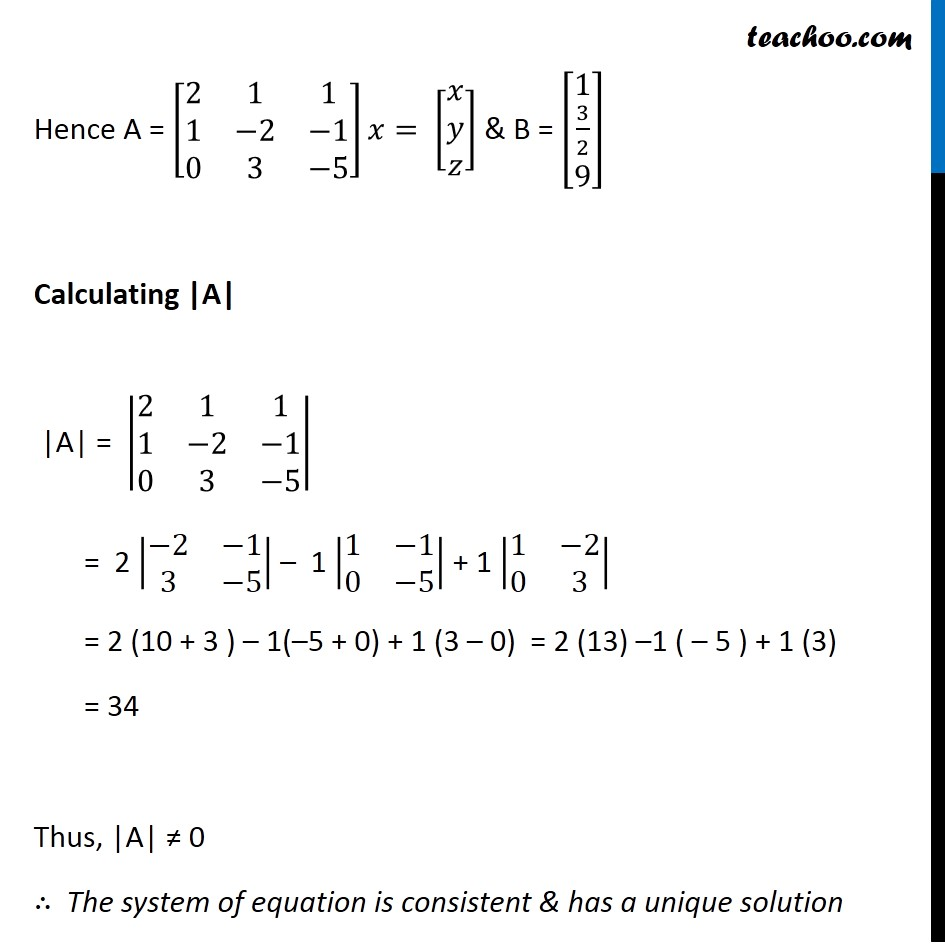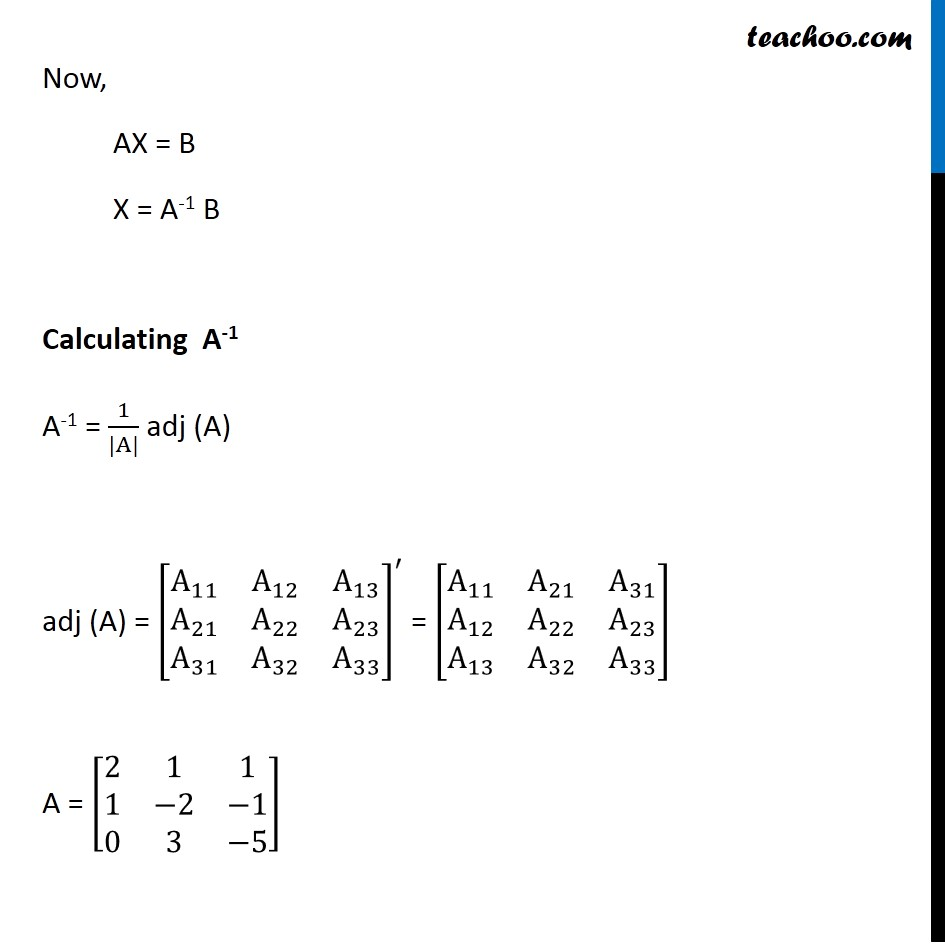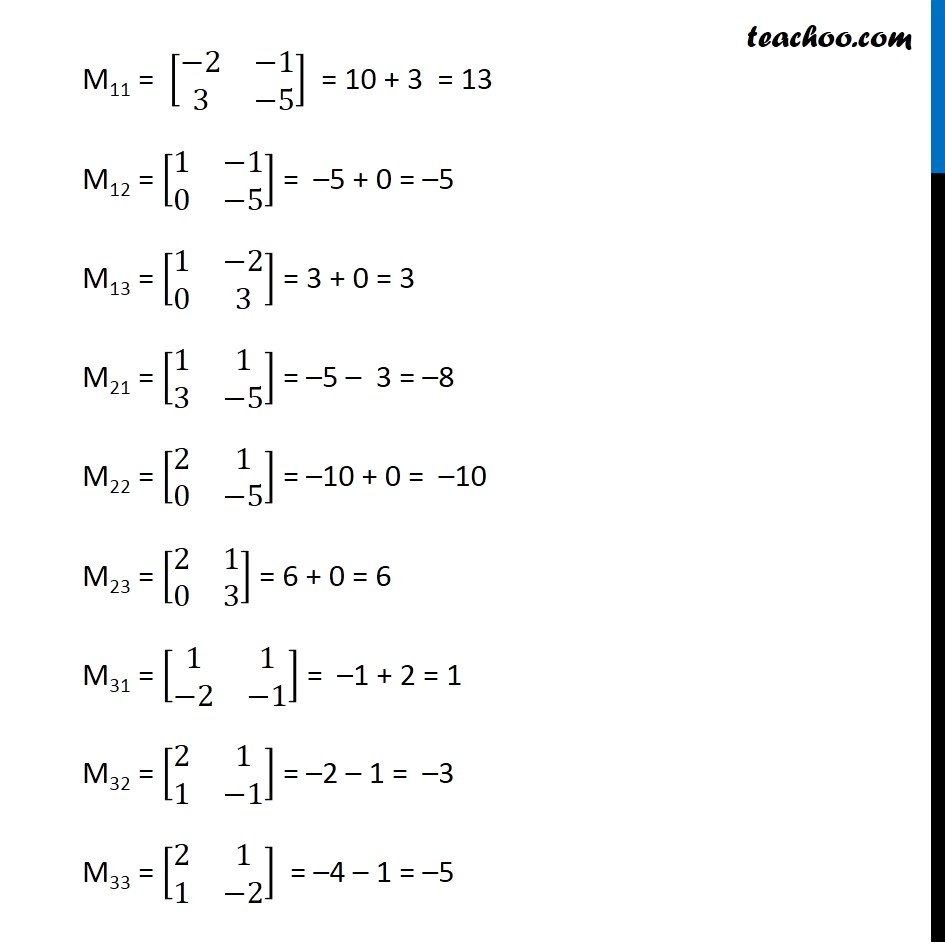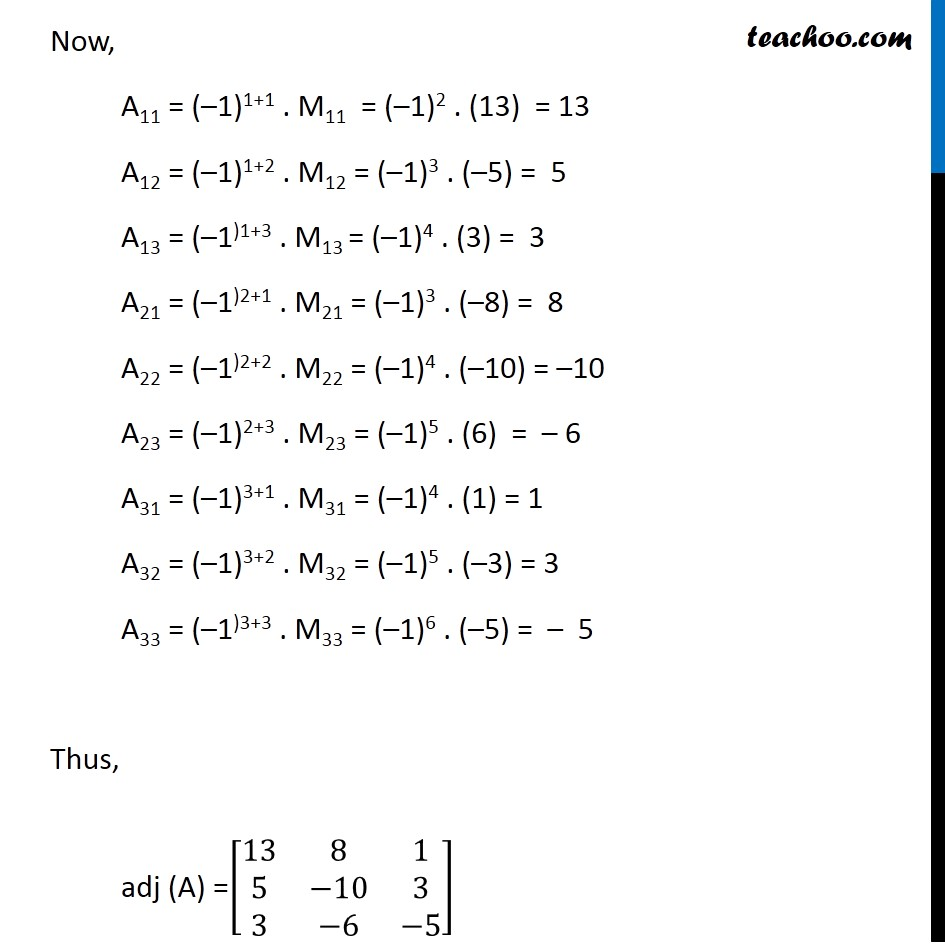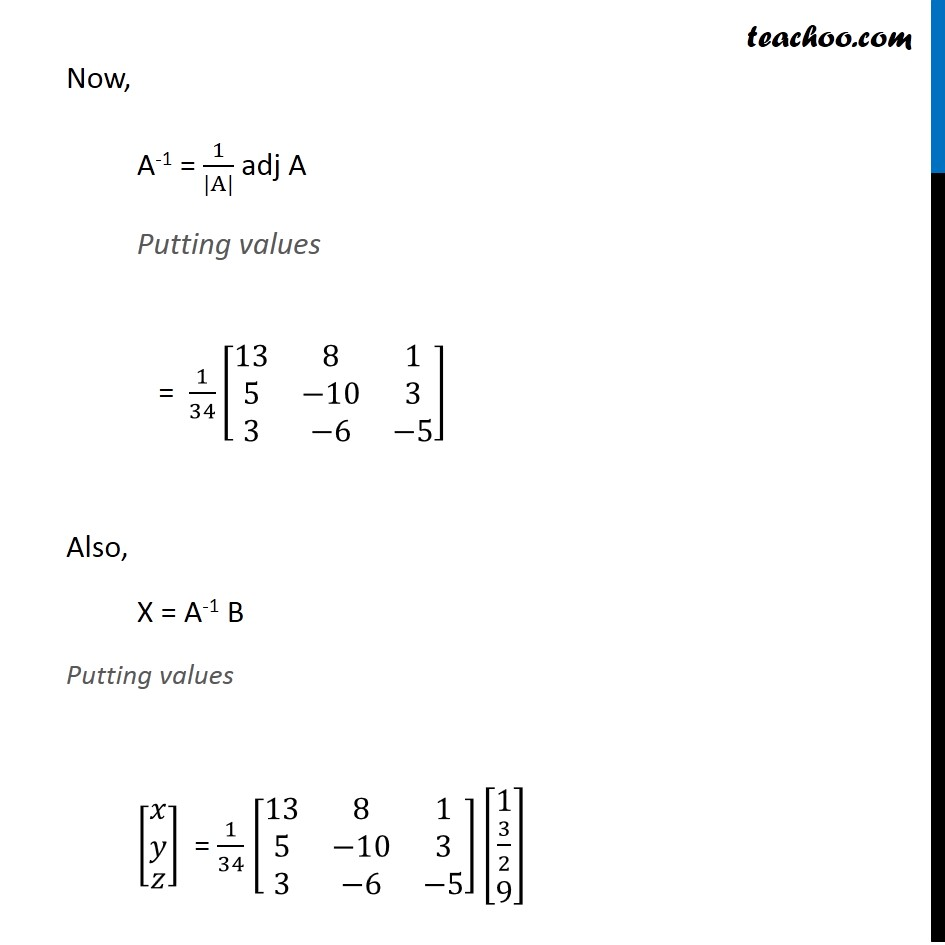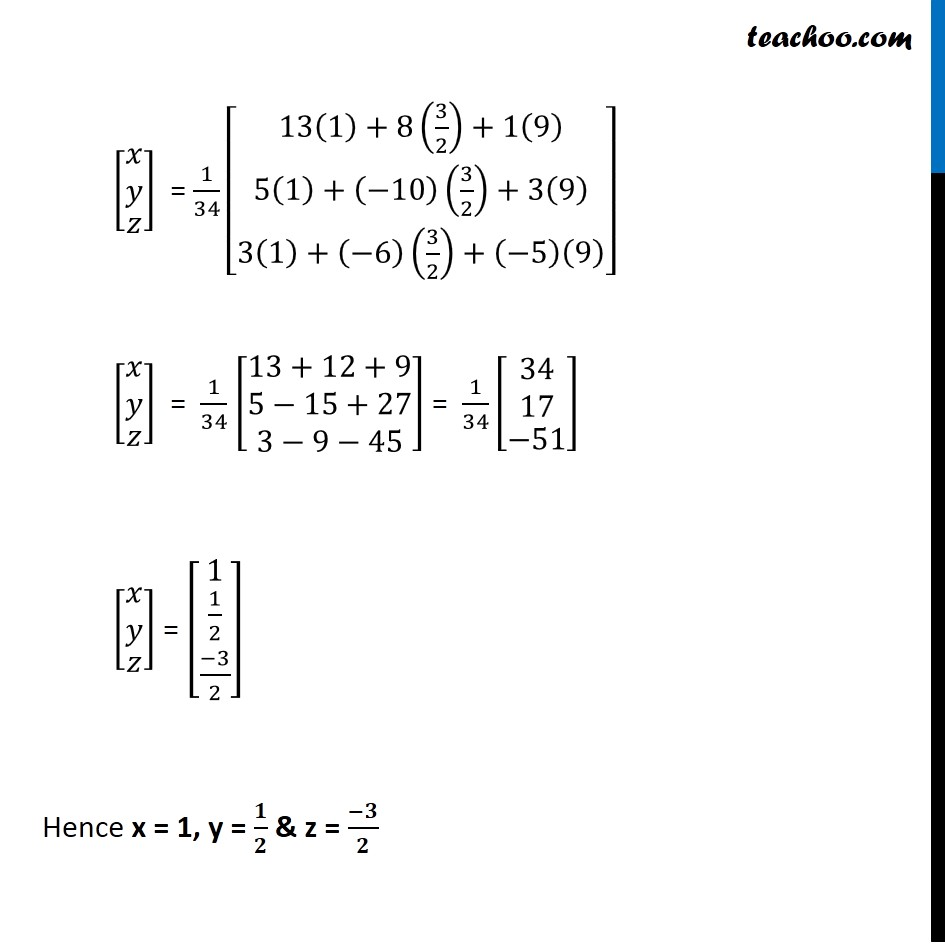Now learn Economics at Teachoo for Class 12

### Transcript

Ex 4.6, 11 Solve system of linear equations, using matrix method. 2x + y + z = 1 x – 2y – z = 3/2 3y – 5z = 9 The system of equation is 2x + y + z = 1 x – 2y – z = 3/2 3y – 5z = 9 Writing above equation as AX = B [■8(2&1&1@1&−2&−1@0&3&−5)][■8(𝑥@𝑦@𝑧)] = [■8(1@3/2@9)] Hence A = [■8(2&1&1@1&−2&−1@0&3&−5)]𝑥= [■8(𝑥@𝑦@𝑧)] & B = [■8(1@3/2@9)] Calculating |A| |A| = |■8(2&1&1@1&−2&−1@0&3&−5)| = 2 |■8(−2&−1@3&−5)| – 1 |■8(1&−1@0&−5)| + 1 |■8(1&−2@0&3)| = 2 (10 + 3 ) – 1(–5 + 0) + 1 (3 – 0) = 2 (13) –1 ( – 5 ) + 1 (3) = 34 Thus, |A| ≠ 0 ∴ The system of equation is consistent & has a unique solution Now, AX = B X = A-1 B Calculating A-1 A-1 = 1/(|A|) adj (A) adj (A) = [■8(A_11&A_12&A_13@A_21&A_22&A_23@A_31&A_32&A_33 )]^′ = [■8(A_11&A_21&A_31@A_12&A_22&A_23@A_13&A_32&A_33 )] A = [■8(2&1&1@1&−2&−1@0&3&−5)] M11 = [■8(−2&−1@3&−5)] = 10 + 3 = 13 M12 = [■8(1&−1@0&−5)] = –5 + 0 = –5 M13 = [■8(1&−2@0&3)] = 3 + 0 = 3 M21 = [■8(1&1@3&−5)] = –5 – 3 = –8 M22 = [■8(2&1@0&−5)] = –10 + 0 = –10 M23 = [■8(2&1@0&3)] = 6 + 0 = 6 M31 = [■8(1&1@−2&−1)] = –1 + 2 = 1 M32 = [■8(2&1@1&−1)] = –2 – 1 = –3 M33 = [■8(2&1@1&−2)] = –4 – 1 = –5 Now, A11 = (–1)1+1 . M11 = (–1)2 . (13) = 13 A12 = (–1)1+2 . M12 = (–1)3 . (–5) = 5 A13 = (–1)1+3 . M13 = (–1)4 . (3) = 3 A21 = (–1)2+1 . M21 = (–1)3 . (–8) = 8 A22 = (–1)2+2 . M22 = (–1)4 . (–10) = –10 A23 = (–1)2+3 . M23 = (–1)5 . (6) = – 6 A31 = (–1)3+1 . M31 = (–1)4 . (1) = 1 A32 = (–1)3+2 . M32 = (–1)5 . (–3) = 3 A33 = (–1)3+3 . M33 = (–1)6 . (–5) = – 5 Thus, adj (A) =[■8(13&8&1@5&−10&3@3&−6&−5)] Now, A-1 = 1/(|A|) adj A Putting values = 1/34 [■8(13&8&1@5&−10&3@3&−6&−5)] Also, X = A-1 B Putting values [█(■8(𝑥@𝑦)@𝑧)] = 1/34 [■8(13&8&1@5&−10&3@3&−6&−5)][█(■8(1@3/2)@9)] [█(■8(𝑥@𝑦)@𝑧)] = 1/34 [■8(13(1)+8(3/2)+1(9)@5(1)+(−10)(3/2)+3(9)@3(1)+(−6)(3/2)+(−5)(9) )] [█(■8(𝑥@𝑦)@𝑧)] = 1/34 [■8(13+12+9@5−15+27@3−9−45)] = 1/34 [█(■8(34@17)@−51)] [█(■8(𝑥@𝑦)@𝑧)] = [█(■8(1@1/2)@(−3)/2)] Hence x = 1, y = 𝟏/𝟐 & z = (−𝟑)/𝟐